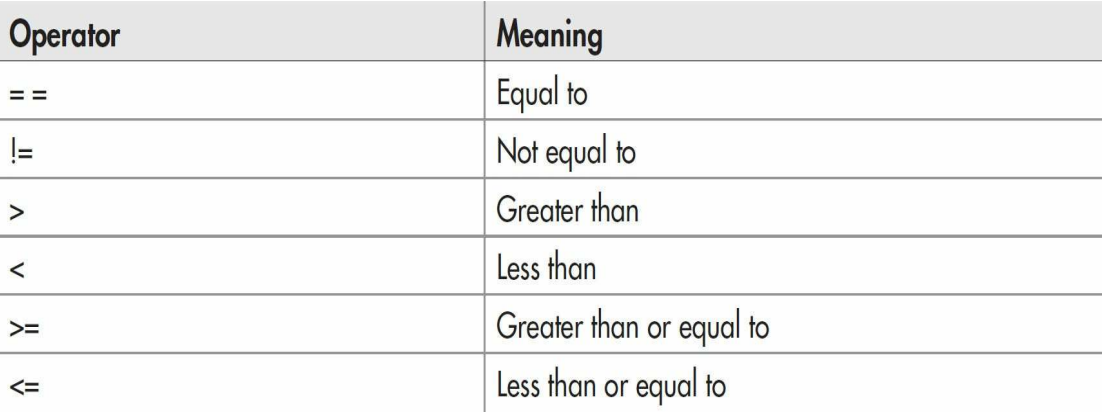Java Comparison Operators

# Java Comparison Operators

Comparison Operator In Java
In the terms relational operator, relational refers to the relationships that values can have with one another. Since the relational operators produce true or false results, they often work with logical operators.
The relational operators are shown here -A relational operator compares two values and is used to determine the relationship between them. For example, != returns true if its two operands are not equal.
The next table summarizes the relational operators, or in other words.
Example-

 import java.util.*; class Bool { public static void main(String[] args) { Random rand = new Random(47); int i = rand.nextInt(100); int j = rand.nextInt(100); print("i = " + i); print("j = " + j); print("i > j is " + (i > j)); print("i < j is " + (i < j)); print("i >= j is " + (i >= j)); print("i <= j is " + (i <= j)); print("i == j is " + (i == j)); print("i != j is " + (i != j));
Output-

 i = 58 j = 55 i > j is true i < j is false i >= j is true i <= j is false i == j is false i != j is true